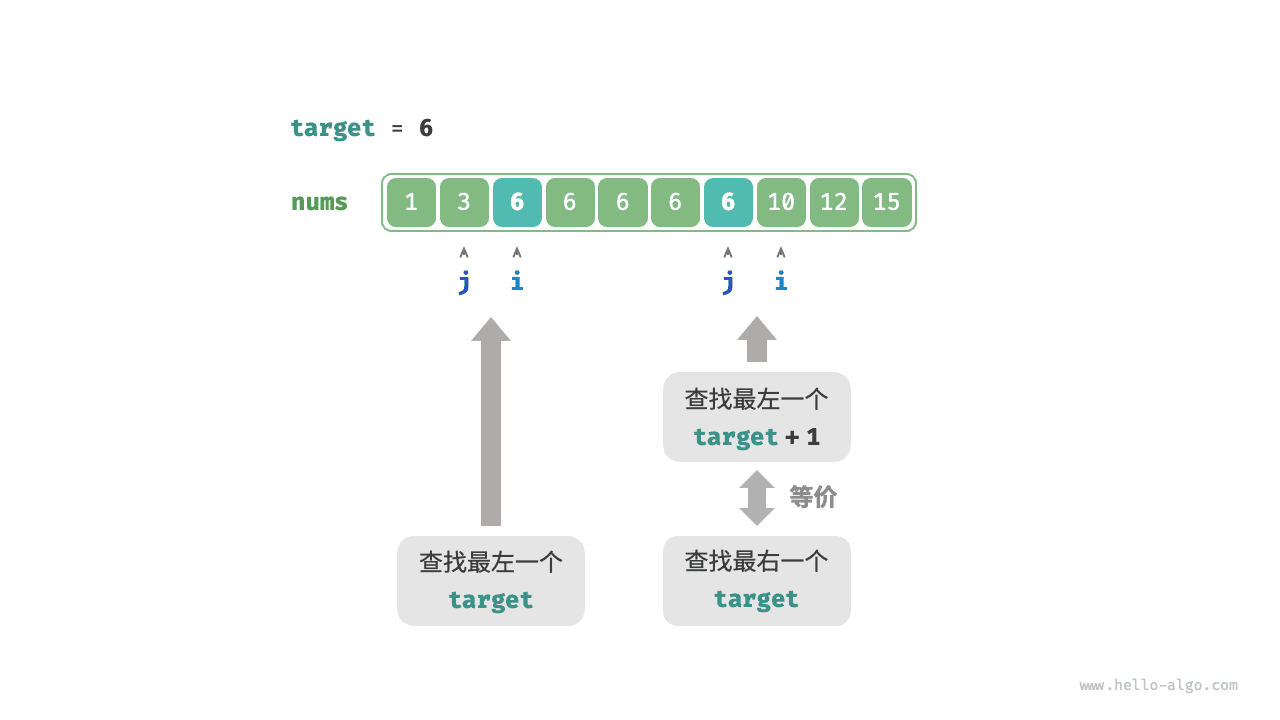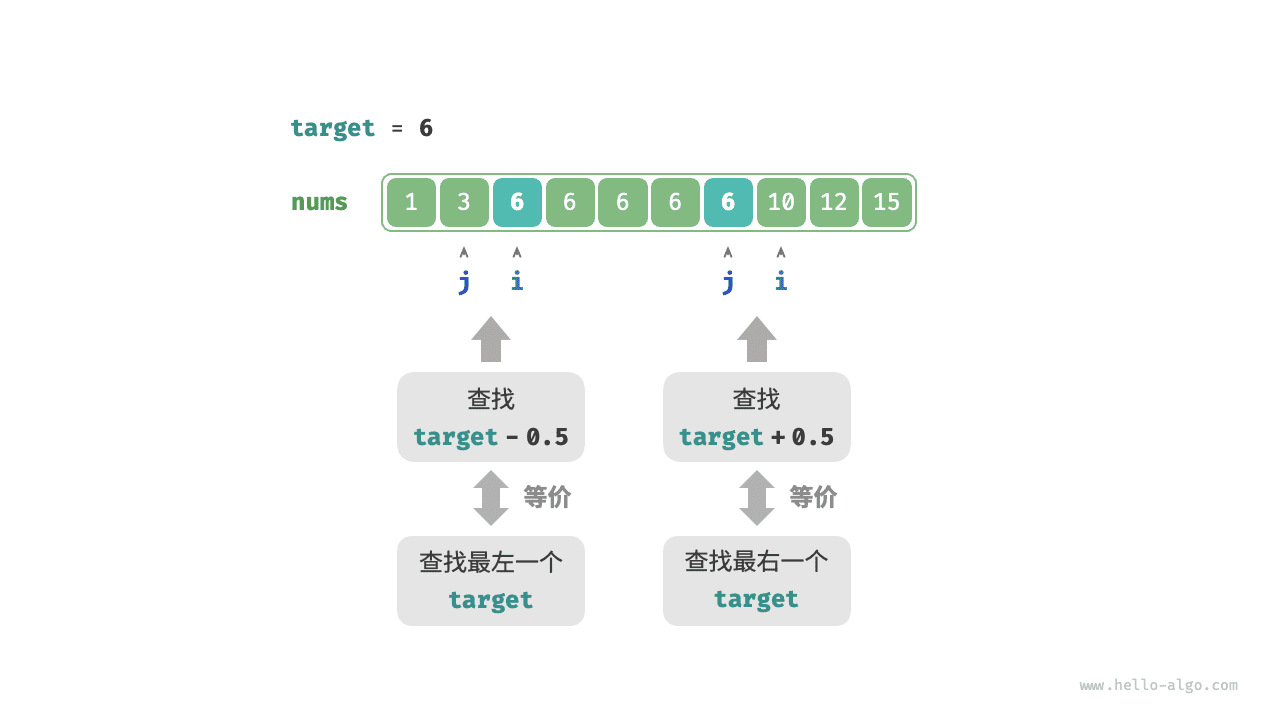# 10.3   二分查找边界¶

## 10.3.1   查找左边界¶

Question

• 插入点的索引 $$i$$ 越界。
• 元素 nums[i]target 不相等。

binary_search_edge.py
def binary_search_left_edge(nums: list[int], target: int) -> int:
"""二分查找最左一个 target"""
# 等价于查找 target 的插入点
i = binary_search_insertion(nums, target)
# 未找到 target ，返回 -1
if i == len(nums) or nums[i] != target:
return -1
# 找到 target ，返回索引 i
return i

binary_search_edge.cpp
/* 二分查找最左一个 target */
int binarySearchLeftEdge(vector<int> &nums, int target) {
// 等价于查找 target 的插入点
int i = binarySearchInsertion(nums, target);
// 未找到 target ，返回 -1
if (i == nums.size() || nums[i] != target) {
return -1;
}
// 找到 target ，返回索引 i
return i;
}

binary_search_edge.java
/* 二分查找最左一个 target */
int binarySearchLeftEdge(int[] nums, int target) {
// 等价于查找 target 的插入点
int i = binary_search_insertion.binarySearchInsertion(nums, target);
// 未找到 target ，返回 -1
if (i == nums.length || nums[i] != target) {
return -1;
}
// 找到 target ，返回索引 i
return i;
}

binary_search_edge.cs
/* 二分查找最左一个 target */
int BinarySearchLeftEdge(int[] nums, int target) {
// 等价于查找 target 的插入点
int i = binary_search_insertion.BinarySearchInsertion(nums, target);
// 未找到 target ，返回 -1
if (i == nums.Length || nums[i] != target) {
return -1;
}
// 找到 target ，返回索引 i
return i;
}

binary_search_edge.go
/* 二分查找最左一个 target */
func binarySearchLeftEdge(nums []int, target int) int {
// 等价于查找 target 的插入点
i := binarySearchInsertion(nums, target)
// 未找到 target ，返回 -1
if i == len(nums) || nums[i] != target {
return -1
}
// 找到 target ，返回索引 i
return i
}

binary_search_edge.swift
/* 二分查找最左一个 target */
func binarySearchLeftEdge(nums: [Int], target: Int) -> Int {
// 等价于查找 target 的插入点
let i = binarySearchInsertion(nums: nums, target: target)
// 未找到 target ，返回 -1
if i == nums.count || nums[i] != target {
return -1
}
// 找到 target ，返回索引 i
return i
}

binary_search_edge.js
/* 二分查找最左一个 target */
function binarySearchLeftEdge(nums, target) {
// 等价于查找 target 的插入点
const i = binarySearchInsertion(nums, target);
// 未找到 target ，返回 -1
if (i === nums.length || nums[i] !== target) {
return -1;
}
// 找到 target ，返回索引 i
return i;
}

binary_search_edge.ts
/* 二分查找最左一个 target */
function binarySearchLeftEdge(nums: Array<number>, target: number): number {
// 等价于查找 target 的插入点
const i = binarySearchInsertion(nums, target);
// 未找到 target ，返回 -1
if (i === nums.length || nums[i] !== target) {
return -1;
}
// 找到 target ，返回索引 i
return i;
}

binary_search_edge.dart
/* 二分查找最左一个 target */
int binarySearchLeftEdge(List<int> nums, int target) {
// 等价于查找 target 的插入点
int i = binarySearchInsertion(nums, target);
// 未找到 target ，返回 -1
if (i == nums.length || nums[i] != target) {
return -1;
}
// 找到 target ，返回索引 i
return i;
}

binary_search_edge.rs
/* 二分查找最左一个 target */
fn binary_search_left_edge(nums: &[i32], target: i32) -> i32 {
// 等价于查找 target 的插入点
let i = binary_search_insertion(nums, target);
// 未找到 target ，返回 -1
if i == nums.len() as i32 || nums[i as usize] != target {
return -1;
}
// 找到 target ，返回索引 i
i
}

binary_search_edge.c
/* 二分查找最左一个 target */
int binarySearchLeftEdge(int *nums, int numSize, int target) {
// 等价于查找 target 的插入点
int i = binarySearchInsertion(nums, numSize, target);
// 未找到 target ，返回 -1
if (i == numSize || nums[i] != target) {
return -1;
}
// 找到 target ，返回索引 i
return i;
}

binary_search_edge.zig
[class]{}-[func]{binarySearchLeftEdge}


## 10.3.2   查找右边界¶

### 1.   复用查找左边界¶binary_search_edge.py
def binary_search_right_edge(nums: list[int], target: int) -> int:
"""二分查找最右一个 target"""
# 转化为查找最左一个 target + 1
i = binary_search_insertion(nums, target + 1)
# j 指向最右一个 target ，i 指向首个大于 target 的元素
j = i - 1
# 未找到 target ，返回 -1
if j == -1 or nums[j] != target:
return -1
# 找到 target ，返回索引 j
return j

binary_search_edge.cpp
/* 二分查找最右一个 target */
int binarySearchRightEdge(vector<int> &nums, int target) {
// 转化为查找最左一个 target + 1
int i = binarySearchInsertion(nums, target + 1);
// j 指向最右一个 target ，i 指向首个大于 target 的元素
int j = i - 1;
// 未找到 target ，返回 -1
if (j == -1 || nums[j] != target) {
return -1;
}
// 找到 target ，返回索引 j
return j;
}

binary_search_edge.java
/* 二分查找最右一个 target */
int binarySearchRightEdge(int[] nums, int target) {
// 转化为查找最左一个 target + 1
int i = binary_search_insertion.binarySearchInsertion(nums, target + 1);
// j 指向最右一个 target ，i 指向首个大于 target 的元素
int j = i - 1;
// 未找到 target ，返回 -1
if (j == -1 || nums[j] != target) {
return -1;
}
// 找到 target ，返回索引 j
return j;
}

binary_search_edge.cs
/* 二分查找最右一个 target */
int BinarySearchRightEdge(int[] nums, int target) {
// 转化为查找最左一个 target + 1
int i = binary_search_insertion.BinarySearchInsertion(nums, target + 1);
// j 指向最右一个 target ，i 指向首个大于 target 的元素
int j = i - 1;
// 未找到 target ，返回 -1
if (j == -1 || nums[j] != target) {
return -1;
}
// 找到 target ，返回索引 j
return j;
}

binary_search_edge.go
/* 二分查找最右一个 target */
func binarySearchRightEdge(nums []int, target int) int {
// 转化为查找最左一个 target + 1
i := binarySearchInsertion(nums, target+1)
// j 指向最右一个 target ，i 指向首个大于 target 的元素
j := i - 1
// 未找到 target ，返回 -1
if j == -1 || nums[j] != target {
return -1
}
// 找到 target ，返回索引 j
return j
}

binary_search_edge.swift
/* 二分查找最右一个 target */
func binarySearchRightEdge(nums: [Int], target: Int) -> Int {
// 转化为查找最左一个 target + 1
let i = binarySearchInsertion(nums: nums, target: target + 1)
// j 指向最右一个 target ，i 指向首个大于 target 的元素
let j = i - 1
// 未找到 target ，返回 -1
if j == -1 || nums[j] != target {
return -1
}
// 找到 target ，返回索引 j
return j
}

binary_search_edge.js
/* 二分查找最右一个 target */
function binarySearchRightEdge(nums, target) {
// 转化为查找最左一个 target + 1
const i = binarySearchInsertion(nums, target + 1);
// j 指向最右一个 target ，i 指向首个大于 target 的元素
const j = i - 1;
// 未找到 target ，返回 -1
if (j === -1 || nums[j] !== target) {
return -1;
}
// 找到 target ，返回索引 j
return j;
}

binary_search_edge.ts
/* 二分查找最右一个 target */
function binarySearchRightEdge(nums: Array<number>, target: number): number {
// 转化为查找最左一个 target + 1
const i = binarySearchInsertion(nums, target + 1);
// j 指向最右一个 target ，i 指向首个大于 target 的元素
const j = i - 1;
// 未找到 target ，返回 -1
if (j === -1 || nums[j] !== target) {
return -1;
}
// 找到 target ，返回索引 j
return j;
}

binary_search_edge.dart
/* 二分查找最右一个 target */
int binarySearchRightEdge(List<int> nums, int target) {
// 转化为查找最左一个 target + 1
int i = binarySearchInsertion(nums, target + 1);
// j 指向最右一个 target ，i 指向首个大于 target 的元素
int j = i - 1;
// 未找到 target ，返回 -1
if (j == -1 || nums[j] != target) {
return -1;
}
// 找到 target ，返回索引 j
return j;
}

binary_search_edge.rs
/* 二分查找最右一个 target */
fn binary_search_right_edge(nums: &[i32], target: i32) -> i32 {
// 转化为查找最左一个 target + 1
let i = binary_search_insertion(nums, target + 1);
// j 指向最右一个 target ，i 指向首个大于 target 的元素
let j = i - 1;
// 未找到 target ，返回 -1
if j == -1 || nums[j as usize] != target {
return -1;
}
// 找到 target ，返回索引 j
j
}

binary_search_edge.c
/* 二分查找最右一个 target */
int binarySearchRightEdge(int *nums, int numSize, int target) {
// 转化为查找最左一个 target + 1
int i = binarySearchInsertion(nums, numSize, target + 1);
// j 指向最右一个 target ，i 指向首个大于 target 的元素
int j = i - 1;
// 未找到 target ，返回 -1
if (j == -1 || nums[j] != target) {
return -1;
}
// 找到 target ，返回索引 j
return j;
}

binary_search_edge.zig
[class]{}-[func]{binarySearchRightEdge}


### 2.   转化为查找元素¶

• 查找最左一个 target ：可以转化为查找 target - 0.5 ，并返回指针 $$i$$
• 查找最右一个 target ：可以转化为查找 target + 0.5 ，并返回指针 $$j$$• 给定数组不包含小数，这意味着我们无须关心如何处理相等的情况。
• 因为该方法引入了小数，所以需要将函数中的变量 target 改为浮点数类型。